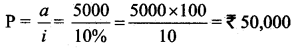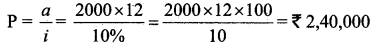Tamilnadu State Board New Syllabus Samcheer Kalvi 11th Business Maths Guide Pdf Chapter 7 Financial Mathematics Ex 7.3 Text Book Back Questions and Answers, Notes.

## Tamilnadu Samacheer Kalvi 11th Business Maths Solutions Chapter 7 Financial Mathematics Ex 7.3

### Samacheer Kalvi 11th Business Maths Financial Mathematics Ex 7.3 Text Book Back Questions and Answers

Question 1.
The dividend received on 200 shares of face value ₹ 100 at 8% dividend value is:
(a) 1600
(b) 1000
(c) 1500
(d) 800
(a) 1600
Hint:
Dividend = 200 × 100 × $$\frac{8}{100}$$ = 1600

Question 2.
What is the amount related is selling 8% stacking 200 shares of face value 100 at 50?
(a) 16,000
(b) 10,000
(c) 7,000
(d) 9,000
(b) 10,000
Hint:
Amount = 200 × 50 = 10000Question 3.
A man purchases a stock of ₹ 20,000 of face value 100 at a premium of 20%, then investment is:
(a) ₹ 20,000
(b) ₹ 25,000
(c) ₹ 22,000
(d) ₹ 30,000
Hint:
Investment = Number of shares × Market value
= $$\frac{20000}{100}$$ × 120
= 24000

Question 4.
A man received a total dividend of ₹ 25,000 at a 10% dividend rate on a stock of face value ₹ 100, then the number of shares purchased.
(a) 3500
(b) 4500
(c) 2500
(d) 300
(c) 2500Question 5.
The brokerage paid by a person on this sale of 400 shares of face value ₹ 100 at 1% brokerage:
(a) ₹ 600
(b) ₹ 500
(c) ₹ 200
(d) ₹ 400
(d) ₹ 400
Hint:
Brokerage = 400 × 100 × $$\frac{1}{100}$$ = ₹ 400

Question 6.
Market price of one share of face value 100 available at a discount of 9½ % with brokerage ½% is:
(a) ₹ 89
(b) ₹ 90
(c) ₹ 91
(d) ₹ 95
(c) ₹ 91
Hint:
Market price = Face value – Discount + Brokerage
= 100 – 9½
= 100 – $$\frac{18}{2}$$
= 100 – 9
= ₹ 91Question 7.
A person brought a 9% stock of face value ₹ 100, for 100 shares at a discount of 10%, then the stock purchased is:
(a) ₹ 9000
(b) ₹ 6000
(c) ₹ 5000
(d) ₹ 4000
(a) ₹ 9000
Hint:
Face value = ₹ 100
Discount = 10%
Market price of a share = 100 – 10 = 90
Number of share = 100
Stock purchased = 100 × 90 = ₹ 9000

Question 8.
The Income on 7 % stock at 80 is:
(a) 9%
(b) 8.75%
(c) 8%
(d) 7%
(b) 8.75%
Hint:
Income = $$\frac{7}{80}$$ × 100
= 0.0875 × 100
= 8.75%Question 9.
The annual income on 500 shares of face value 100 at 15% is:
(a) ₹ 7500
(b) ₹ 5000
(c) ₹ 8000
(d) ₹ 8500
(a) ₹ 7500
Hint:
Income = $$\frac{n \times r \times F . V}{100}$$
= 500 × $$\frac{15}{100}$$ × 100
= ₹ 7500

Question 10.
₹ 5000 is paid as perpetual annuity every year and the rate of C.I. 10%. Then the present value P of an immediate annuity is:
(a) ₹ 60,000
(b) ₹ 50,000
(c) ₹ 10,000
(d) ₹ 80,000
(b) ₹ 50,000
Hint:Question 11.
If ‘a’ is the annual payment, ‘n’ is the number of periods and ‘i’ is compound interest for ₹ 1 then future amount of the annuity is:
(a) A = $$\frac{a}{i}$$ (1 + i) [(1 + i)n – 1]
(b) A = $$\frac{a}{i}$$ [(1 + i)n – 1]
(c) P = $$\frac{a}{i}$$
(d) P = $$\frac{a}{i}$$ (1 + i) [1 – (1 + i)-n]
(b) A = $$\frac{a}{i}$$ [(1 + i)n – 1]

Question 12.
A invested some money in 10% stock at 96. If B wants to invest in an equally good 12% stock, he must purchase a stock worth of:
(a) ₹ 80
(b) ₹ 115.20
(c) ₹ 120
(d) ₹ 125.40
(a) ₹ 80
Hint:
Let x be B stock worth.
Then x × $$\frac{12}{100}$$ = $$\frac{10}{100}$$ × 96
x × 12 = 10 × 96
x = 80Question 13.
An annuity in which payments are made at the beginning of each payment period is called:
(a) Annuity due
(b) An immediate annuity
(c) perpetual annuity
(d) none of these
Annuity due

Question 14.
The present value of the perpetual annuity of ₹ 2000 paid monthly at 10 % compound interest is:
(a) ₹ 2,40,000
(b) ₹ 6,00,000
(c) ₹ 20,40,000
(d) ₹ 2,00,400
(a) ₹ 2,40,000
Hint:Question 15.
An example of a contingent annuity is: